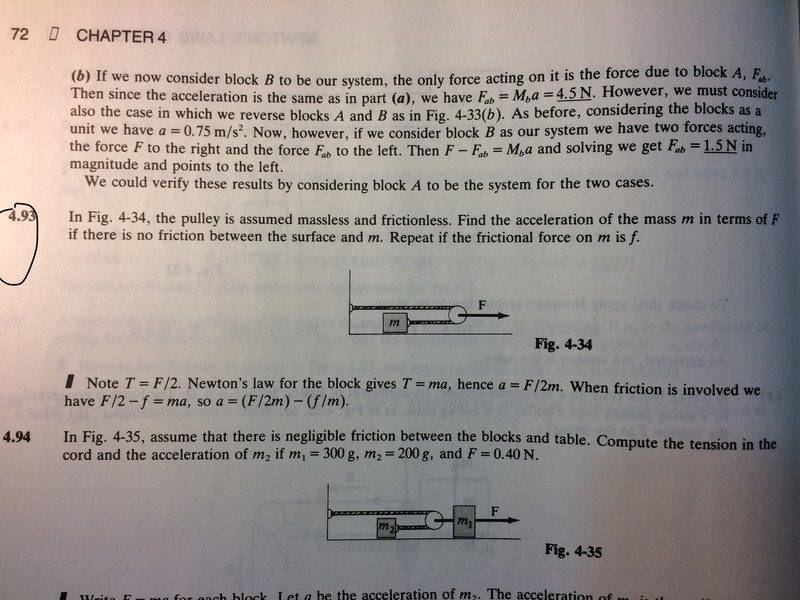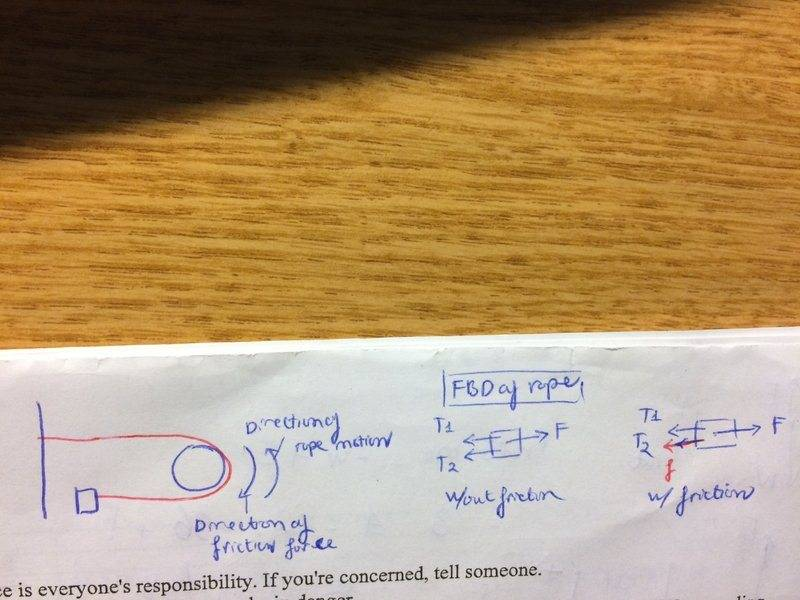# Newton’s Law of Motions: tension forces in a pulley

## Homework Statement

Problem: 4.93[/B]
The pulley is assumed massless and frictionless. The mass of the object attached to the pulley is given in terms of m, the force applied to the pulley is F (refer to diagram), and frictional force is f.

Question: Find the acceleration of the mass m in terms of F if there is no friction between the surface and m. Repeat if the frictional force on m is f.

aceleration=F/2m. (without friction)
acceleration=(F/2m)-(f/m) (with friction)

Diagram:## Homework Equations

F=ma (Newton's Second Law)
Fnet (horizontal)= -f+F (Calculating net force)

## The Attempt at a Solution

My initial answer was similar to the key, except that I forget that the tension of the string attached to the mass is half of the force F. I get that the tension is less than the force F because there are two separate strings doing the entire force F, but I don't know why each is exactly half of F; it seems simple but I could not understand its behavior physically. My question: how do we know T= F/2? Why wouldn’t the tension of the side attached to the wall be greater than the tension attached to the mass, because the mass is probably less heavy and is movable?

Thank you!

#### Attachments

stockzahn
Homework Helper
Gold Member
Just draw a FBD of the rope alone. Since there is no friction between rope and pulley, the tension must be the same in each part of the rope - it is not diminished by a friction force. If the tension in the upper part of the rope and the lower part of the rope is identical, it must be ##F/2##.

Thank you ! That makes sense, I didn't think of making a FBD for the rope. To doublecheck my thinking: so if there is friction on the pulley, the tension force of the rope attached to the box would be lesser than the tension attached to the wall (because the friction force would point along the tension attached to the mass)?
My FBD is like this :Thank you!

#### Attachments

•stockzahn
stockzahn
Homework Helper
Gold Member
To doublecheck my thinking: so if there is friction on the pulley, the tension force of the rope attached to the box would be lesser than the tension attached to the wall (because the friction force would point along the tension attached to the mass)?

That's it. In many of these cases it helps to imagine an "extreme" situation. If for example the bearing of the pulley would be stuck and it doesen't rotate ...

I see. Thanks a lot, I'll have to try that tip!